test 9 section 3 number 18

This one is all about how translations (shifts up, down, left, or right) work in function notation. So, a quick review. If you know what any random functionlooks like, then you know:

•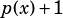will shift that function UP one unit
•will shift that function DOWN one unit
•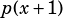will shift that function LEFT one unit
•will shift that function RIGHT one unit

In this question, we’re shown the graph of, and then we’re told that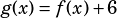. In other words, the graph of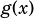will be the same as the graph of, just shifted up 6 units.

We can see from the graph we’re given that the maximum value of function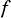is 2, so the maximum value of functionwill be 6 higher than 2: 8!